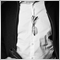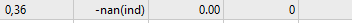# Custom Criteria Coding issues - page 3380

Nicholas C Weber #:

I guess that answers my last reply. LOL. Thanks again. I'm normalizing the crud out of it now. I've revised this EA soooo many times. on the market, it's like 2.6, but on my computer it's well over 20-30. I think it's 2.9.5 or something.

no worries, good luck2606

I would like to point out, if you are comparing prices, you will need to build yourself a NormalizePrice-Function of some sort.

Using NormalizeDouble is insufficieint when comparing prices.

Please check the forum on that topic, as it has been discussed in depth.2606

Nicholas C Weber #:

So this isn't overkill?

`NormalizeDouble(min_dd, 4) = (NormalizeDouble(1.0, 1) / NormalizeDouble(min_dd, 4) );`

This is more than required, think about the accuracy you need. - I would guess this is sufficient already:

`min_dd = NormalizeDouble(1.0 / min_dd, 4);`

As said, it is required to distinguish between a double value and a price value.263

now I'm getting -nan(ind) as a result when there are zero trades. here's what the code looks like now:

```double OnTester()
{
double  param = 0.0;

//  Balance Max + Min Drawdown + Profit Trades Number + Profit Factor + Recovery Factor + Win Ratio:

double  balance = TesterStatistics(STAT_PROFIT);
NormalizeDouble(balance, 2);

double  min_dd = (TesterStatistics(STAT_EQUITY_DD) + TesterStatistics(STAT_BALANCE_DD) / 2);
NormalizeDouble(min_dd, 3);
if(min_dd > 0.0)
{
min_dd = (1 / min_dd);
}
else if(min_dd == 0.0)
{
min_dd = (1);
}

{
}

double equity_dd = TesterStatistics(STAT_EQUITY_DD);
NormalizeDouble(equity_dd, 2);

double balance_dd = TesterStatistics(STAT_BALANCE_DD);
NormalizeDouble(balance_dd, 2);

double profit_factor = TesterStatistics(STAT_PROFIT_FACTOR);
NormalizeDouble(profit_factor, 2);
if(profit_factor > 0.0)
{
profit_factor = (NormalizeDouble(profit_factor, 2));
}
else if(
(profit_factor == 0.0)
&& ((((1 / equity_dd) + (1 / balance_dd)) / 2) < 2.5)
&& (balance > 0.0)
)
{
profit_factor = (500);
}

double recovery_factor = TesterStatistics(STAT_RECOVERY_FACTOR);
NormalizeDouble(recovery_factor, 2);

double expected_profit = TesterStatistics(STAT_EXPECTED_PAYOFF);
NormalizeDouble(expected_profit, 2);

NormalizeDouble(win_ratio, 3);
if(win_ratio < 2)
{
win_ratio = (win_ratio / 10.0);
}
else if(win_ratio > 2)
{
win_ratio = (win_ratio);
}
else if(
(win_ratio == 0.0)
&& (profit_factor == 0.0)
&& (((equity_dd + balance_dd) / 2) < 2.5)
&& (balance > 0.0)
&& (expected_profit > 10)
)
{
win_ratio = (10);
}
else if(win_ratio < 1.0)
{
win_ratio = (NormalizeDouble(win_ratio, 3) / 10);
}

param = ((NormalizeDouble(balance_dd, 2) * NormalizeDouble(min_dd, 2) * NormalizeDouble(profit_trades, 2) * NormalizeDouble(win_ratio, 3) * ((NormalizeDouble(profit_factor, 2) + NormalizeDouble(recovery_factor, 2)) / 2)) / 10);
NormalizeDouble(param, 2);

return(NormalizeDouble(param, 2));
}```

zero trades and trades with zero losses aka zero profit factor now all convert the result from a decimal to "-nan(ind)

I've tried everything.. removing normalize, adding it, removing settings, changing, adding, etc.380

Nicholas C Weber #:

now I'm getting -nan(ind) as a result when there are zero trades. here's what the code looks like now:

zero trades and trades with zero losses aka zero profit factor now all convert the result from a decimal to "-nan(ind)

I've tried everything.. removing normalize, adding it, removing settings, changing, adding, etc.

Oh no... The code is certainly looking more intricate from where we started - bugs have the habit of creeping in as the complexity grows

Where are you getting NaN? Can you pinpoint which variable?

Also I note you are using different decimal places in different places - better to be consistent. It is easy to define a constant and re-use in every place - that is one thing to try.

```#define def_decimals 5

double  balance = TesterStatistics(STAT_PROFIT);
NormalizeDouble(balance, def_decimals);

```
The key recommendation is to be careful when comparing doubles and use NormalizeDouble() to ensure both values have the same accuracy, beyond that its your choice if you want to normalize every double, I usually leave them as is and just normalize when comparing.263

R4tna C #:

Where are you getting NaN? Can you pinpoint which variable?

Also I note you are using different decimal places in different places - better to be consistent.

It is easy to define a constant and re-use in every place - try that first and see if it helps

Here's the new code. I think it was a few issues. I'm about to test it. I changed all the normalize to 2 decimals. I canged balance/equity_dd to ***_dd_percent, as I was comparing it to < 2.5. I'm just error code goofin'. "How could this happen?!"

```double OnTester()
{
double  param = 0.0;

//  Balance Max + Min Drawdown + Profit Trades Number + Profit Factor + Recovery Factor + Win Ratio:

double  balance = TesterStatistics(STAT_PROFIT);

{
}

double equity_dd = TesterStatistics(STAT_EQUITY_DD);

double balance_dd = TesterStatistics(STAT_BALANCE_DD);

double  min_dd = ((NormalizeDouble(equity_dd, 2) + NormalizeDouble(balance_dd, 2)) / 2);
NormalizeDouble(min_dd, 2);
if(NormalizeDouble(min_dd, 2) > 0.0)
{
min_dd = (1 / NormalizeDouble(min_dd, 2));
}
else if(NormalizeDouble(min_dd, 2) == 0.0)
{
min_dd = (1);
}

double equity_dd_percent = TesterStatistics(STAT_EQUITYDD_PERCENT);
double balance_dd_percent = TesterStatistics(STAT_BALANCEDD_PERCENT);

double profit_factor = TesterStatistics(STAT_PROFIT_FACTOR);
if(NormalizeDouble(profit_factor, 2) > 0.0)
{
profit_factor = (NormalizeDouble(profit_factor, 2));
}
else if(
(NormalizeDouble(profit_factor, 2) == 0.0)
&& (((NormalizeDouble(equity_dd_percent, 2) + NormalizeDouble(balance_dd, 2)) / 2) < 2.5)
&& (NormalizeDouble(balance, 2) > 0.0)
)
{
profit_factor = (500);
}

double recovery_factor = TesterStatistics(STAT_RECOVERY_FACTOR);
NormalizeDouble(recovery_factor, 2);

double expected_profit = TesterStatistics(STAT_EXPECTED_PAYOFF);
NormalizeDouble(expected_profit, 2);

NormalizeDouble(win_ratio, 2);
if(NormalizeDouble(win_ratio, 2) < 2)
{
win_ratio = (NormalizeDouble(win_ratio, 2) / 10.0);
}
else if(NormalizeDouble(win_ratio, 2) > 2)
{
win_ratio = (NormalizeDouble(win_ratio, 2));
}
else if(
(NormalizeDouble(win_ratio, 2) == 0.0)
&& (NormalizeDouble(profit_factor, 2) == 0.0)
&& (((NormalizeDouble(equity_dd_percent, 2) + NormalizeDouble(balance_dd, 2)) / 2) < 2.5)
&& (NormalizeDouble(balance, 2) > 0.0)
&& (NormalizeDouble(expected_profit, 2) > 10)
)
{
win_ratio = (10);
}
else if(NormalizeDouble(win_ratio, 2) < 1.0)
{
win_ratio = (NormalizeDouble(win_ratio, 2) / 10);
}

param = ((NormalizeDouble(balance, 2) * NormalizeDouble(min_dd, 2) * NormalizeDouble(profit_trades, 2) * (NormalizeDouble(win_ratio, 2) * (NormalizeDouble(profit_factor, 2) + NormalizeDouble(recovery_factor, 2)))) / 10);
NormalizeDouble(param, 2);

return(NormalizeDouble(param, 2));
}```
I also changed the calculation from multiplying win_ratio against the whole formula to just the profit and recovery factors.263-nan(ind)

metatrader updated twice in the past few hours. maybe that's what it is. idk. never seen it before.2606

I dont want to disturb your error findings, but the usage of Normalize double like shown here is non-sense.

```double  min_dd = ((NormalizeDouble(equity_dd, 2) + NormalizeDouble(balance_dd, 2)) / 2);
NormalizeDouble(min_dd, 2);```

As said, do it like so:

```double  min_dd = NormalizeDouble((equity_dd + balance_dd) / 2.0, 2);
```

Edit, and by the way, dont call it every time you access the variable, cal lit once, and save the result....

`double min_dd = NormalizeDouble(min_dd, 2);`

Then later in ypur code, every time you assign a new value, use your NormalizeDouble... - You are overkilling it at the moment.380

Nicholas C Weber #:

-nan(ind)

metatrader updated twice in the past few hours. maybe that's what it is. idk. never seen it before.

I was starting to look at your code but have had to rush to the hospital for a family medical emergency.

Will resume when possible380

R4tna C #:
I was starting to look at your code but have had to rush to the hospital for a family medical emergency.

Will resume when possible
The key recommendation is to use Normalize at the time of comparison.

I think I missed that your doing it more frequently as Dominik has pointed out

Anyway let's resume when time allows